SLVS676D JUNE   2006  – July 2015

PRODUCTION DATA.

1. Features
2. Applications
3. Description
4. Revision History
5. Device Comparison Table
6. Pin Configuration and Functions
7. Specifications
8. Detailed Description
1. 8.1 Overview
2. 8.2 Functional Block Diagram
3. 8.3 Feature Description
4. 8.4 Device Functional Modes
5. 8.5 Programming
1. 8.5.1 EasyScale™ Interface: One-Pin Serial Interface for Dynamic Output Voltage Adjustment
6. 8.6 Register Maps
9. Application and Implementation
1. 9.1 Application Information
1. 9.1.1 Output Voltage Setting
2. 9.2 Typical Applications
1. 9.2.1 Typical Application Circuit 1.5-V and 2.85-V Adjustable Outputs
1. 9.2.1.1 Design Requirements
2. 9.2.1.2 Detailed Design Procedure
1. 9.2.1.2.1 Output Filter Design (Inductor and Output Capacitor)
3. 9.2.1.3 Application Curves
2. 9.2.2 Typical Application Circuit TPS62421
10. 10Power Supply Recommendations
11. 11Layout
12. 12Device and Documentation Support
13. 13Mechanical, Packaging, and Orderable Information

• DRC|10
• DRC|10

## 9 Application and Implementation

NOTE

Information in the following applications sections is not part of the TI component specification, and TI does not warrant its accuracy or completeness. TI’s customers are responsible for determining suitability of components for their purposes. Customers should validate and test their design implementation to confirm system functionality.

### 9.1 Application Information

#### 9.1.1.1 Converter 1 Adjustable Default Output Voltage Setting

The output voltage can be calculated to:

Equation 4.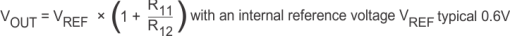To keep the operating current to a minimum, TI recommends selecting R12 within a range of 180 kΩ to 360 kΩ. The sum of R12 and R11 should not exceed ~1 MΩ. For higher output voltages than 3.3 V, TI recommends choosing lower values than 180 kΩ for R12. Route the DEF_1 line away from noise sources, such as the inductor or the SW1 line. The FB1 line needs to be directly connected to the output capacitor. An internal feed-forward capacitor is connected to this pin, therefore there is no need for an external feed-forward capacitor for converter 1.

#### 9.1.1.2 Converter 2

The default output voltage of converter 2 can be set by an external resistor network. For converter 2 the same recommendations apply as for converter 1. In addition to that, a 33-pF external feed-forward capacitor Cff2 for good load transient response must be used.

The output voltage can be calculated to:

Equation 5.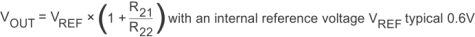Route the ADJ2 line away from noise sources, such as the inductor or the SW2 line. In case the interface is used for converter 2, connect ADJ2 pin directly to VOUT2

### 9.2 Typical Applications

#### 9.2.1 Typical Application Circuit 1.5-V and 2.85-V Adjustable Outputs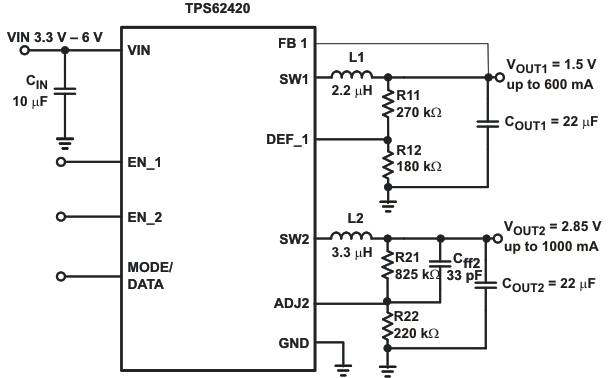Figure 14. Typical Application Circuit 1.5-V and 2.85-V Adjustable Outputs

#### 9.2.1.1 Design Requirements

The step-down converter design can be adapted to different output voltage and load current needs by choosing external components appropriate. The following design procedure is adequate for whole VIN, VOUT and load current range of TPS62420.

#### 9.2.1.2.1 Output Filter Design (Inductor and Output Capacitor)

The device is optimized to operate with inductors of 2.2 μH to 4.7 μH and output capacitors of 10 μF to 22 μF.

For operation with a 2.2-μH inductor, a 22-μF capacitor is suggested.

#### 9.2.1.2.1.1 Inductor Selection

The selected inductor has to be rated for its DC resistance and saturation current. The DC resistance of the inductance will influence directly the efficiency of the converter. Therefore an inductor with lowest DC resistance should be selected for highest efficiency.

Equation 6 calculates the maximum inductor current under static load conditions. The saturation current of the inductor should be rated higher than the maximum inductor current as calculated with Equation 7. This is recommended because during heavy load transient the inductor current will rise above the calculated value.

Equation 6.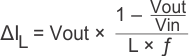Equation 7.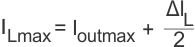where

• f = Switching frequency (2.25 MHz typical)
• L = Inductor value
• ΔIL= Peak-to-peak inductor ripple current
• ILmax = Maximum inductor current

The highest inductor current will occur at maximum VIN.

Open core inductors have a soft saturation characteristic and they can usually handle higher inductor currents versus a comparable shielded inductor.

A more conservative approach is to select the inductor current rating just for the maximum switch current of the corresponding converter. It must be considered, that the core material from inductor to inductor differs and will have an impact on the efficiency especially at high switching frequencies.

Refer to Table 7 and the typical applications for possible inductors.

### Table 7. List of Inductors

DIMENSIONS [mm3] INDUCTOR TYPE SUPPLIER
3.2 × 2.6 × 1.0 MIPW3226 FDK
3 × 3 × 0.9 LPS3010 Coilcraft
2.8 × 2.6 × 1.0 VLF3010 TDK
2.8 x 2.6 × 1.4 VLF3014 TDK
3 × 3 × 1.4 LPS3015 Coilcraft
3.9 × 3.9 × 1.7 LPS4018 Coilcraft

#### 9.2.1.2.1.2 Output Capacitor Selection

The advanced fast response voltage mode control scheme of the two converters allows the use of small ceramic capacitors with a typical value of 10 μF, without having large output voltage undershoots and overshoots during heavy load transients. Ceramic X7R/X5R capacitors having low ESR values result in lowest output voltage ripple and are therefore recommended.

If ceramic output capacitors are used, the capacitor RMS ripple current rating will always meet the application requirements. The RMS ripple current is calculated as:

Equation 8.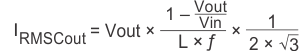At nominal load current the inductive converters operate in PWM mode and the overall output voltage ripple is the sum of the voltage spike caused by the output capacitor ESR plus the voltage ripple caused by charging and discharging the output capacitor:

Equation 9.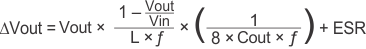Where the highest output voltage ripple occurs at the highest input voltage VIN.

At light load currents the converters operate in power-save mode and the output voltage ripple is dependent on the output capacitor value. The output voltage ripple is set by the internal comparator delay and the external capacitor. Higher output capacitors like 22-μF values minimize the voltage ripple in PFM mode and tighten DC output accuracy in PFM mode.

#### 9.2.1.2.1.3 Input Capacitor Selection

Because of the nature of the buck converter having a pulsating input current, a low-ESR input capacitor is required for best input voltage filtering and minimizing the interference with other circuits caused by high input voltage spikes. The converters need a ceramic input capacitor of 10 μF. The input capacitor can be increased without any limit for better input voltage filtering.

#### 9.2.1.3 Application Curves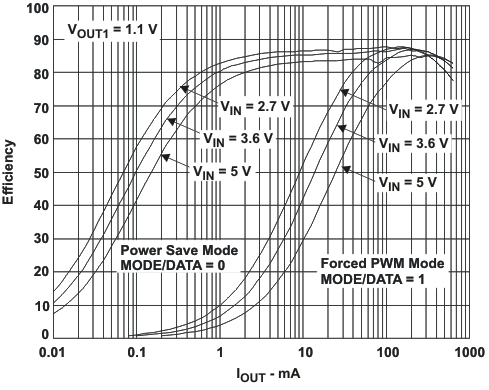Figure 15. Efficiency VOUT1 = 1.1 V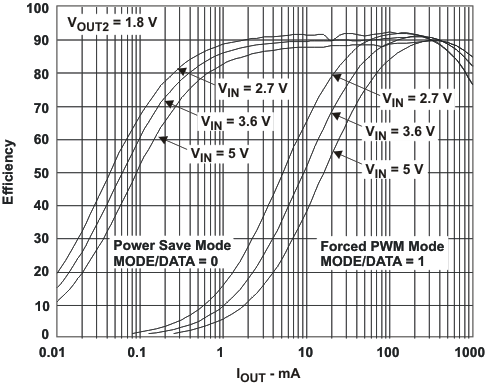Figure 17. Efficiency VOUT2 = 1.8 V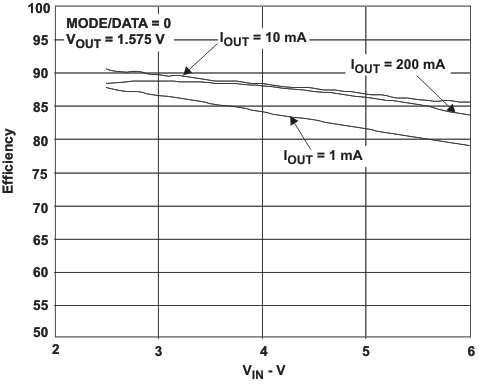Figure 19. Efficiency vs VIN , VOUT1 = 1.575 V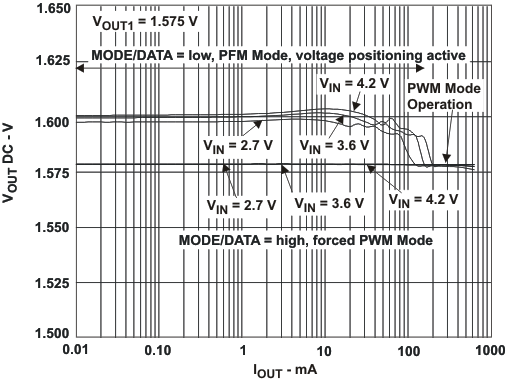Figure 21. DC Output Accuracy VOUT1 = 1.575 V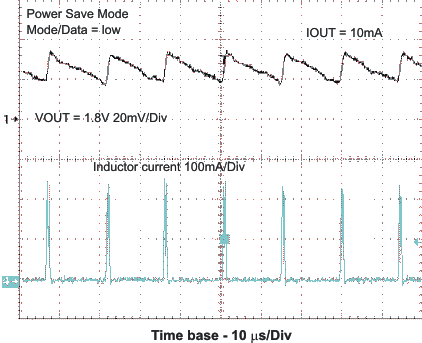Figure 23. Light Load Output Voltage Ripple
in Power-Save Mode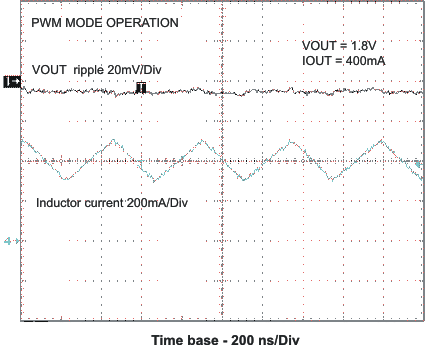Figure 25. Output Voltage Ripple
in PWM Mode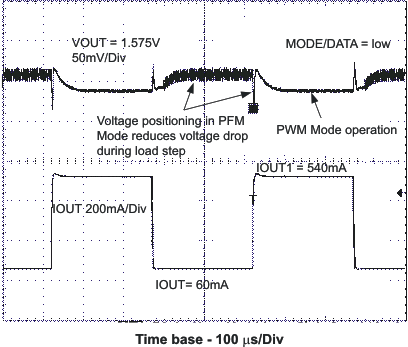Figure 27. Load Transient Response PFM/PWM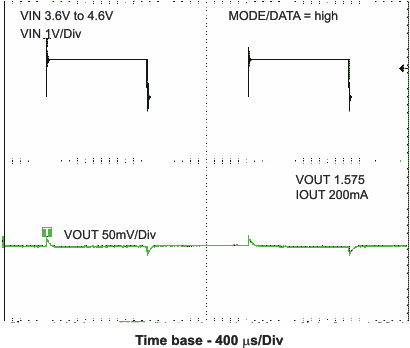Figure 29. Line Transient Response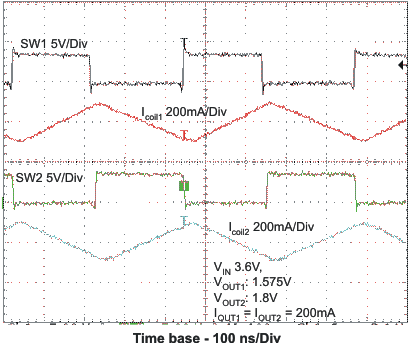Figure 31. Typical Operation VIN = 3.6 V,
VOUT1 = 1.575 V, VOUT2 = 1.8 V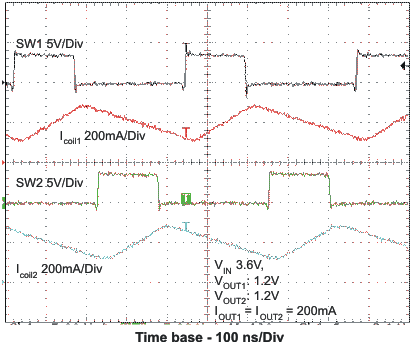Figure 33. Typical Operation VIN = 3.6 V,
VOUT1 = 1.2 V, VOUT2 = 1.2 V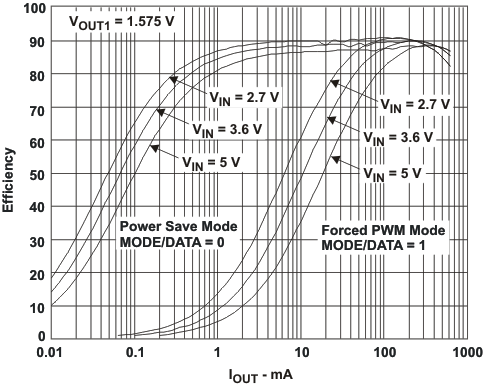Figure 16. Efficiency VOUT1 = 1.575 V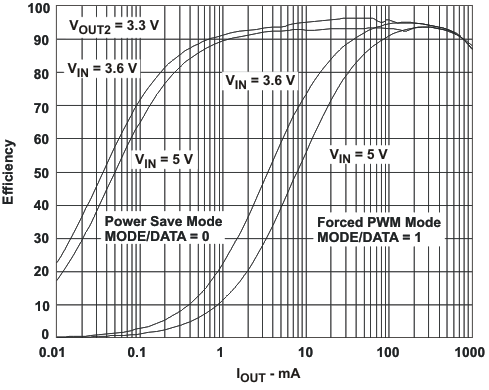Figure 18. Efficiency VOUT2 = 3.3 VFigure 20. Efficiency vs VIN, VOUT2 = 3.3 V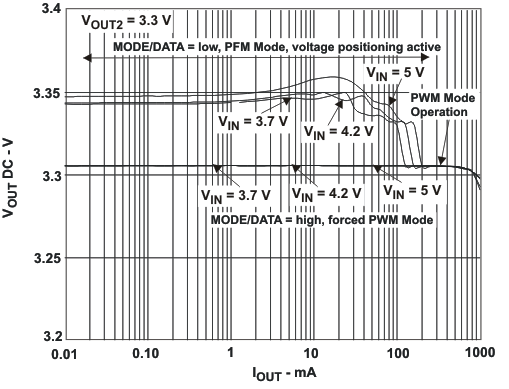Figure 22. DC Output Accuracy VOUT2 = 3.3 V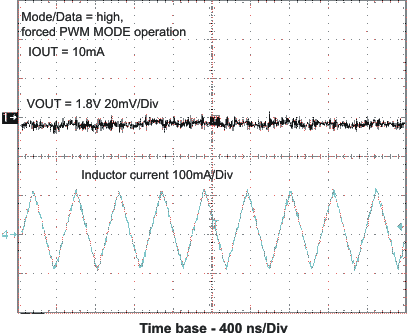Figure 24. Output Voltage Ripple in Forced PWM Mode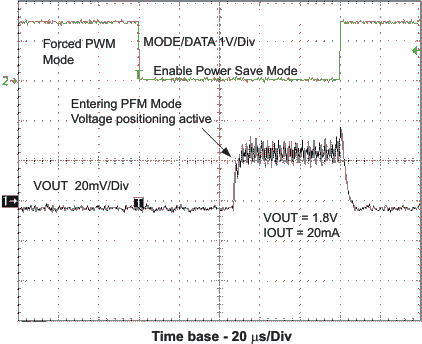Figure 26. Forced PWM/PFM Mode Transition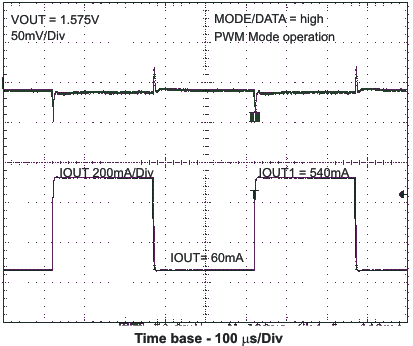Figure 28. Load Transient Response PWM OperationFigure 30. Start-Up Timing One Converter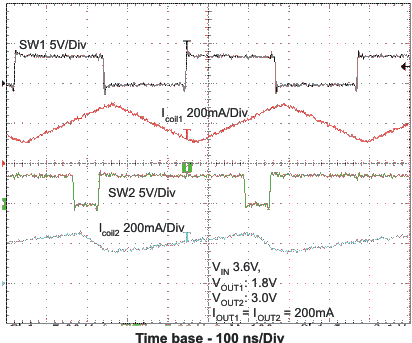Figure 32. Typical Operation VIN = 3.6 V,
VOUT1 = 1.8 V, VOUT2 = 3 V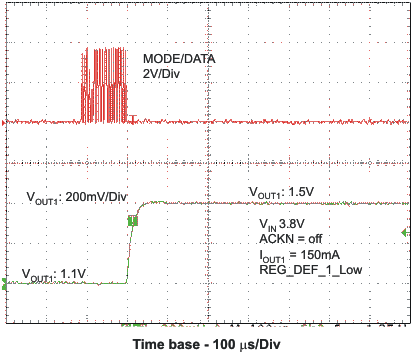Figure 34. VOUT1 Change With EasyScale™ Interface

#### 9.2.2 Typical Application Circuit TPS62421

In fixed output voltage version TPS62421, the default output voltage of converter 1 is fixed to 1.2 V or 1.8 V depending on the DEF_1 pin level. The default output voltage of converter 2 is fixed to 1.8 V. The ADJ2 pin must be connected directly to the converter 2 output voltage.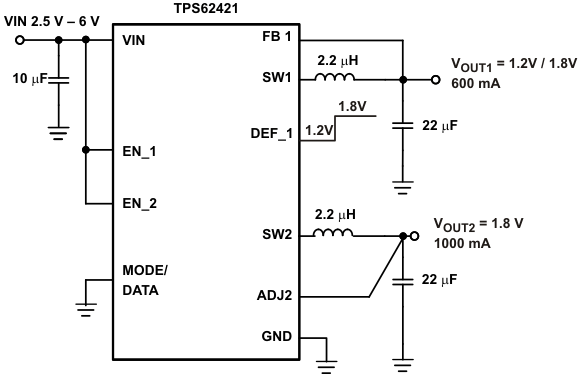Figure 35. Typical Application Circuit TPS62421